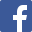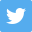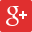1930
Senior Section P W D L F A GD GA Pt Adj Reserve Section Isthmian League `1.` `Wimbledon` `26` `18` `6` `2` `69` `37` `32` `1.86` `42` `2.` `Dulwich Hamlet` `26` `12` `9` `5` `51` `39` `12` `1.31` `33` `3.` `Wycombe Wanderers` `26` `12` `6` `8` `67` `45` `22` `1.49` `30` `4.` `The Casuals` `26` `12` `6` `8` `71` `56` `15` `1.27` `30` `5.` `St. Albans City` `26` `11` `7` `8` `67` `66` `1` `1.02` `29` `6.` `Ilford` `26` `10` `6` `10` `70` `62` `8` `1.13` `26` `7.` `Oxford City` `26` `10` `5` `11` `43` `48` `-5` `0.90` `25` `8.` `London Caledonians` `26` `8` `8` `10` `43` `53` `-10` `0.81` `24` `9.` `Kingstonian` `26` `10` `4` `12` `49` `64` `-15` `0.77` `24` `10.` `Tufnell Park` `26` `9` `5` `12` `45` `61` `-16` `0.74` `23` `11.` `Nunhead` `26` `9` `4` `13` `49` `54` `-5` `0.91` `22` `12.` `Woking` `26` `9` `4` `13` `56` `63` `-7` `0.89` `22` `13.` `Clapton` `26` `7` `4` `15` `62` `75` `-13` `0.83` `18` `14.` `Leytonstone` `26` `6` `4` `16` `46` `65` `-19` `0.71` `16` ` ` `364` `143` `78` `143` `788` `788` `0` `364` `0` Isthmian League `1.` `Dulwich Hamlet (reserves)` `26` `20` `4` `2` `107` `39` `68` `2.74` `44` `2.` `The Casuals (reserves)` `26` `16` `3` `7` `74` `46` `28` `1.61` `35` `3.` `Wimbledon (reserves)` `26` `14` `4` `8` `83` `63` `20` `1.32` `32` `4.` `Nunhead (reserves)` `26` `14` `3` `9` `78` `63` `15` `1.24` `31` `5.` `Oxford City (reserves)` `26` `14` `1` `11` `85` `63` `22` `1.35` `29` `6.` `St. Albans City (reserves)` `26` `10` `8` `8` `63` `57` `6` `1.11` `28` `7.` `London Caledonians (reserves)` `26` `9` `7` `10` `69` `58` `11` `1.19` `25` `8.` `Tufnell Park (reserves)` `26` `9` `4` `13` `61` `67` `-6` `0.91` `22` `9.` `Clapton (reserves)` `26` `10` `2` `14` `59` `79` `-20` `0.75` `22` `10.` `Woking (reserves)` `26` `9` `4` `13` `61` `87` `-26` `0.70` `22` `11.` `Leytonstone (reserves)` `26` `10` `2` `14` `47` `78` `-31` `0.60` `22` `12.` `Ilford (reserves)` `26` `8` `3` `15` `69` `73` `-4` `0.95` `19` `13.` `Kingstonian (reserves)` `26` `9` `1` `16` `63` `81` `-18` `0.78` `19` `14.` `Wycombe Wanderers (reserves)` `26` `6` `2` `18` `49` `114` `-65` `0.43` `14` ` ` `364` `158` `48` `158` `968` `968` `0` `364` `0`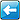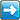This page has been visited 1813 times. The contents of this site are (c) their respective owners. If you wish to use anything within, please check their status first. If you have any information, photographs, comments, additions, memorabilia or corrections to this site please contact us